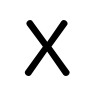+0

# If there are 800000 tickets, and I use 2000 tickets, what is the % of me winning?

0
602
5

If there are 800000 tickets, and I use 2000 tickets, what is the % of me winning?

Jul 9, 2015

#2
0

If there is only ONE winning ticket, when you buy 1 ticket you have a 1/800000 chance of winning.  Buy another ticket from the 79999 tickets remaining and you have a 1/79999 chance of winning....etc

So    1/800000 + 1/799999 + 1/799998 ..........1/798001   =I think!    Can anyone 2nd that?
Jul 9, 2015
#3
0

=Jul 9, 2015
#4
0
Sorry,   as 'Anonymous' poster it wouldn't let me post by 'pasting' the answer, but here it is

= .002503128651703143....
Jul 9, 2015
#6
0

If there is one winning ticket then you have 2000 chances to win out of a possible 800000 tickets.

$${\frac{{\mathtt{2\,000}}}{{\mathtt{800\,000}}}}{\mathtt{\,\times\,}}{\mathtt{100}} = {\frac{{\mathtt{1}}}{{\mathtt{4}}}} = {\mathtt{0.25}}$$

That is 0.25% chance     (exactly 1 chance in 400)

Jul 10, 2015